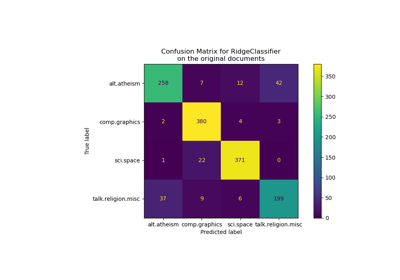# sklearn.linear_model.RidgeClassifier¶

class sklearn.linear_model.RidgeClassifier(alpha=1.0, *, fit_intercept=True, normalize=False, copy_X=True, max_iter=None, tol=0.001, class_weight=None, solver='auto', random_state=None)[source]

Classifier using Ridge regression.

This classifier first converts the target values into {-1, 1} and then treats the problem as a regression task (multi-output regression in the multiclass case).

Read more in the User Guide.

Parameters
alphafloat, default=1.0

Regularization strength; must be a positive float. Regularization improves the conditioning of the problem and reduces the variance of the estimates. Larger values specify stronger regularization. Alpha corresponds to 1 / (2C) in other linear models such as LogisticRegression or sklearn.svm.LinearSVC.

fit_interceptbool, default=True

Whether to calculate the intercept for this model. If set to false, no intercept will be used in calculations (e.g. data is expected to be already centered).

normalizebool, default=False

This parameter is ignored when fit_intercept is set to False. If True, the regressors X will be normalized before regression by subtracting the mean and dividing by the l2-norm. If you wish to standardize, please use sklearn.preprocessing.StandardScaler before calling fit on an estimator with normalize=False.

copy_Xbool, default=True

If True, X will be copied; else, it may be overwritten.

max_iterint, default=None

Maximum number of iterations for conjugate gradient solver. The default value is determined by scipy.sparse.linalg.

tolfloat, default=1e-3

Precision of the solution.

class_weightdict or ‘balanced’, default=None

Weights associated with classes in the form {class_label: weight}. If not given, all classes are supposed to have weight one.

The “balanced” mode uses the values of y to automatically adjust weights inversely proportional to class frequencies in the input data as n_samples / (n_classes * np.bincount(y)).

solver{‘auto’, ‘svd’, ‘cholesky’, ‘lsqr’, ‘sparse_cg’, ‘sag’, ‘saga’}, default=’auto’

Solver to use in the computational routines:

• ‘auto’ chooses the solver automatically based on the type of data.

• ‘svd’ uses a Singular Value Decomposition of X to compute the Ridge coefficients. More stable for singular matrices than ‘cholesky’.

• ‘cholesky’ uses the standard scipy.linalg.solve function to obtain a closed-form solution.

• ‘sparse_cg’ uses the conjugate gradient solver as found in scipy.sparse.linalg.cg. As an iterative algorithm, this solver is more appropriate than ‘cholesky’ for large-scale data (possibility to set tol and max_iter).

• ‘lsqr’ uses the dedicated regularized least-squares routine scipy.sparse.linalg.lsqr. It is the fastest and uses an iterative procedure.

• ‘sag’ uses a Stochastic Average Gradient descent, and ‘saga’ uses its unbiased and more flexible version named SAGA. Both methods use an iterative procedure, and are often faster than other solvers when both n_samples and n_features are large. Note that ‘sag’ and ‘saga’ fast convergence is only guaranteed on features with approximately the same scale. You can preprocess the data with a scaler from sklearn.preprocessing.

New in version 0.17: Stochastic Average Gradient descent solver.

New in version 0.19: SAGA solver.

random_stateint, RandomState instance, default=None

Used when solver == ‘sag’ or ‘saga’ to shuffle the data. See Glossary for details.

Attributes
coef_ndarray of shape (1, n_features) or (n_classes, n_features)

Coefficient of the features in the decision function.

coef_ is of shape (1, n_features) when the given problem is binary.

intercept_float or ndarray of shape (n_targets,)

Independent term in decision function. Set to 0.0 if fit_intercept = False.

n_iter_None or ndarray of shape (n_targets,)

Actual number of iterations for each target. Available only for sag and lsqr solvers. Other solvers will return None.

classes_ndarray of shape (n_classes,)

The classes labels.

Ridge

Ridge regression.

RidgeClassifierCV

Ridge classifier with built-in cross validation.

Notes

For multi-class classification, n_class classifiers are trained in a one-versus-all approach. Concretely, this is implemented by taking advantage of the multi-variate response support in Ridge.

Examples

>>> from sklearn.datasets import load_breast_cancer
>>> from sklearn.linear_model import RidgeClassifier
>>> clf = RidgeClassifier().fit(X, y)
>>> clf.score(X, y)
0.9595...


Methods

 Predict confidence scores for samples. fit(X, y[, sample_weight]) Fit Ridge classifier model. get_params([deep]) Get parameters for this estimator. Predict class labels for samples in X. score(X, y[, sample_weight]) Return the mean accuracy on the given test data and labels. set_params(**params) Set the parameters of this estimator.
__init__(alpha=1.0, *, fit_intercept=True, normalize=False, copy_X=True, max_iter=None, tol=0.001, class_weight=None, solver='auto', random_state=None)[source]

Initialize self. See help(type(self)) for accurate signature.

decision_function(X)[source]

Predict confidence scores for samples.

The confidence score for a sample is the signed distance of that sample to the hyperplane.

Parameters
Xarray_like or sparse matrix, shape (n_samples, n_features)

Samples.

Returns
array, shape=(n_samples,) if n_classes == 2 else (n_samples, n_classes)

Confidence scores per (sample, class) combination. In the binary case, confidence score for self.classes_ where >0 means this class would be predicted.

fit(X, y, sample_weight=None)[source]

Fit Ridge classifier model.

Parameters
X{ndarray, sparse matrix} of shape (n_samples, n_features)

Training data.

yndarray of shape (n_samples,)

Target values.

sample_weightfloat or ndarray of shape (n_samples,), default=None

Individual weights for each sample. If given a float, every sample will have the same weight.

New in version 0.17: sample_weight support to Classifier.

Returns
selfobject

Instance of the estimator.

get_params(deep=True)[source]

Get parameters for this estimator.

Parameters
deepbool, default=True

If True, will return the parameters for this estimator and contained subobjects that are estimators.

Returns
paramsmapping of string to any

Parameter names mapped to their values.

predict(X)[source]

Predict class labels for samples in X.

Parameters
Xarray_like or sparse matrix, shape (n_samples, n_features)

Samples.

Returns
Carray, shape [n_samples]

Predicted class label per sample.

score(X, y, sample_weight=None)[source]

Return the mean accuracy on the given test data and labels.

In multi-label classification, this is the subset accuracy which is a harsh metric since you require for each sample that each label set be correctly predicted.

Parameters
Xarray-like of shape (n_samples, n_features)

Test samples.

yarray-like of shape (n_samples,) or (n_samples, n_outputs)

True labels for X.

sample_weightarray-like of shape (n_samples,), default=None

Sample weights.

Returns
scorefloat

Mean accuracy of self.predict(X) wrt. y.

set_params(**params)[source]

Set the parameters of this estimator.

The method works on simple estimators as well as on nested objects (such as pipelines). The latter have parameters of the form <component>__<parameter> so that it’s possible to update each component of a nested object.

Parameters
**paramsdict

Estimator parameters.

Returns
selfobject

Estimator instance.

## Examples using sklearn.linear_model.RidgeClassifier¶